﻿ Two Dimensional Hotelling Model with Dirichlet Boundary ConditionJournal of Game Theory

p-ISSN: 2325-0046    e-ISSN: 2325-0054

2018;  7(1): 1-6

doi:10.5923/j.jgt.20180701.01### Two Dimensional Hotelling Model with Dirichlet Boundary Condition

Yunus Emre Ozcan1, Bahriye Karaca2, Veysel Ulusoy3

1Department of International Finance, Yeditepe University, Istanbul, Turkey

2Faculty of Economics and Administrative Sciences, Gelisim University, Istanbul, Turkey

3Faculty of Applied Sciences, Yeditepe University, Istanbul, Turkey

Correspondence to: Yunus Emre Ozcan, Department of International Finance, Yeditepe University, Istanbul, Turkey.
 Email: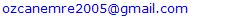Abstract

This paper examines the location equilibrium in spatial competition framework with two dimensional spaces inside a unit disk. We drive the general conditions for location function under the indirect utility function of consumers. We found the unique solution of symmetric location problem with Dirichlet boundary condition in complex plane for a pin point.

Keywords: Spatial Competition, Location Choice, Game Theory

Cite this paper: Yunus Emre Ozcan, Bahriye Karaca, Veysel Ulusoy, Two Dimensional Hotelling Model with Dirichlet Boundary Condition, Journal of Game Theory, Vol. 7 No. 1, 2018, pp. 1-6. doi: 10.5923/j.jgt.20180701.01.

### 1. Introduction

The analysis of firms’ competition is one of the main aspects of the economic studies. The problem of firms’ competition has a vast literature and the classical concepts such as perfect competition, price equilibrium and oligopoly have already been introduced. One of the important concepts of competition literature, Bertrand paradox1 states that firms earn zero profit due to price reduction by its’ competitors to attract more customers in the market until the marginal cost of product equals the product price for each firm in the market. Product differentiation, transportation cost, non-perfect competition concepts relax this assumption.
Location game is the main focused area of this paper; this concept was first introduced to economic literature by . Hotelling model analyzes the behavior of two sellers of a homogenous product who chooses price and location in a bounded one dimensional marketplace where consumers are distributed on line length l and product price is associated with transportation cost which is proportional to the distance between the consumers and firms . In this setting, the optimal location choice of the firms is the main driver of the spatial competition amongst the firms. In this original article; it has been found that competing firms tend to be located close to each other and price equilibrium does not exist unless one of the competing firms chooses quadratic transportation cost rather than linear transportation cost. Linear transportation cost could not provide an existence of price equilibrium for each possible pair of locations in one dimensional market place .
In , Hotelling model was generalized to find locational existence equilibrium over a disk for spatial competition.2 Economides  showed that regions of existence of equilibrium in the price game for intermediate product differentiation with quadratic and linear transportation cost.3
Tabuchi  has demonstrated that competing two firms locate at the midpoint of one side opposite to each other in rectangular two dimensional market spaces. On the other hand, he stated that firms tend to minimize their distance in one dimension market place. In the case of a hypercube market place, Irmen and Thisse  have shown that two firms to differentiate their products in one dimension only (see e.g ). Feldin  has shown that two firms location on the perimeter of the disk and firms located themselves exactly opposite of each other. In case of different shapes of the market without the spatial restriction, firms can locate the outside of the market areas (see e.g ).
Our methodological framework allows us to examine firm’s location problem inside of a unit disk. We try to solve competing firm’s symmetric optimal location problem in order to find out existence of Nash equilibrium on a location game.
Main contribution of the article to existing literature is to set the Dirichlet boundary conditions for two-dimensional market place in terms of optimal location problem for spatial competition framework. Consumers are uniformly distributed inside of a unit disc rather than on an interval. Consumers have the same demand, preferences and income. Transportation cost is homogenous and increases with distance. Consumers’ objective is to have maximum utility. We present the indirect consumer’s utility function by using complex analysis and having producers distributed inside the unit disc.
In the first part of the paper, the necessary material from complex analysis is reviewed. Second part of the paper states the theoretical model and third part of the paper explains symmetric optimal location problem for existence of equilibrium with the boundary conditions.

### 2. Background

This section gives insight on the Dirichlet boundary value problems for complex partial differential equations. Basic definitions and concepts from complex analysis are presented.
Definition 3.1 : Let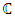complex plane, a complex valued function of the variable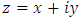and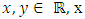is called the real part of z and is denoted by Rez, y is called imaginary part of z and is denoted by Imz. The complex number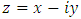is called the conjugate of z. The extended complex plane is denoted by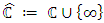, a domain D in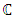is called regular if it is bounded and its boundary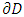is being a smooth curve.
A complex valued function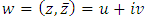be defined in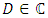and let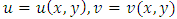are real valued functions. When u and v are differentiable and w is independent of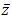in an open set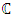the function w is called analytic in the set.
A complex valued function w which is independent of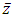, satisfying the differential equation in an open domain D in C,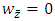is called analytic function in D.
The complex partial differential operator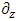and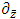are defined by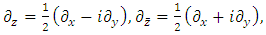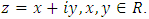Theorem 3.2  Let w be an analytic function in a simply connected domain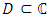and let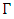be a simple closed curve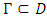, then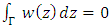The fundamental tools for solving the boundary value problems for complex partial differential equations are the Gauss theorem and the Cauchy -Pompeiu Representation formula.
Theorem 3.3  (Gauss Theorem, complex form) Let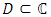be a regular domain and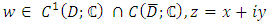, then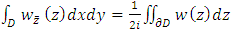and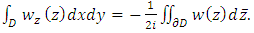From the Gauss Theorem the Cauchy-Pompeiu Representation can be obtained.
Theorem 3.4  (Cauchy- Pompeiu Representation) Let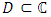be a regular domain and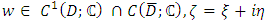, then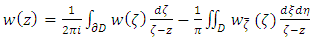and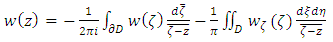### 3. Brief description of the Model

This section gives a brief description of the model described in this paper. We consider a unit disk market4 with two dimensional space in which two competing firms exist and both firms offer identical products in all respects except for the location of availability and firms choose locations inside the unit disk. Consumers are uniformly distributed inside the unit disk. Each consumer demands a single unit of product and faces transportation cost to buy one unit of product. Under these settings, we explore the perfect Nash equilibrium.

### 4. Model and Its Equilibrium

By definition spatial market considers equilibrium; none of the competing firms find a combination of location and price that makes one firm more profitable than another. Given by this definition, this market consists of two competing firms; a and b, and the consumers choose between the two and their utility can be expressed as follows;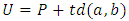(1)
Where P is denoted as price and is constant, d is denoted as the location of firms and t is the transportation cost. Transportation cost increases linearly as the distance between consumer and the location increase. Customers are seeking the minimization of their cost with respect to their transportation cost. Since we are not considering the price game, The Nash location equilibrium can be defined as no firm in the game want to relocate itself. This is defined by5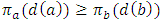(2)
First approach of the model is to find out the optimal location for firm a inside the unit disk in order to be more attractive to the consumers by reducing consumers’ transportation cost. Throughout this study D will be unit disc.
Proposition 1: firm location is a continuous function on the boundary of the unit disc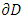and twice differentiable. Firms are apart from each other at equal and opposite distance from consumers.
Proof.
We define the location problem as the complex valued function to find optimal interior point in a unit disk.
Location of firm a is given by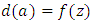where;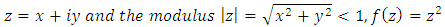Symmetric solution of the location equilibrium is the complex conjugate of the location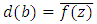where;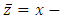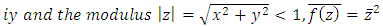Theorem 5.1 . The Dirichlet problem for the inhomogeneous Bitsadze equation in the unit disc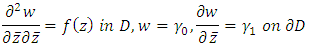for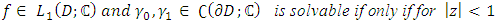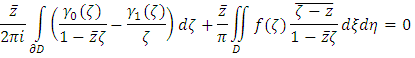and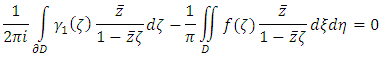the solution then is uniquely given by the formula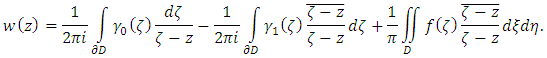As an application of Theorem 5.1 considering the following boundary value problem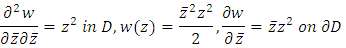Cauchy-Pompeiu Representation reduces the boundary value problem for differential equation to integral equation in order to prove the existence of uniqueness.
The first solvability condition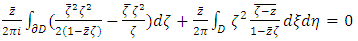(3)
and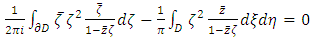(4)
the solution is given by formula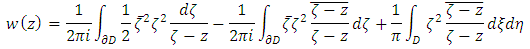By using the Gauss Theorem, the equation 3 i.e. first solvability condition can be written as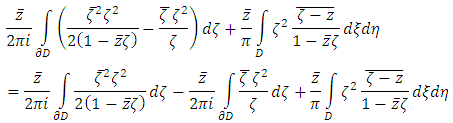And from the Gauss Theorem it follows that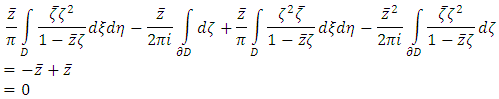Using Gauss Theorem the second solvability condition can be written as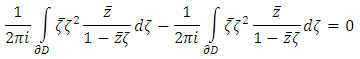So the solution is uniquely given by the following formula.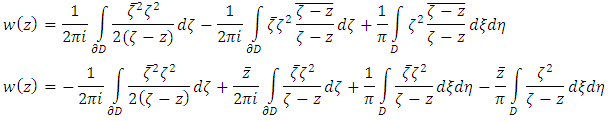Using Cauchy-Pompeiu Representation formula it follows that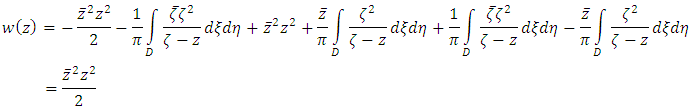This is the firm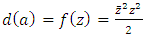optimal location inside the unit disk given boundary conditions. It is always possible for firm b to increase its profit by moving to the closest location of firm a and cutting its price to just below that of firm a. Therefore stability of equilibrium cannot emerge. We employ same procedure for firm b so that no firm can make a small change in its location and price which generate a larger gross profit than that obtained by its present choice of location and price. At this stage, we try to find optimal location for firm b by using complex conjugate of the location of firm a.
Theorem 5.2  The Dirichlet problem for complex conjugate of the inhomogeneous Bitsadze equation in unit disk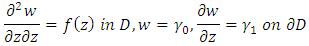for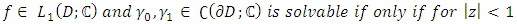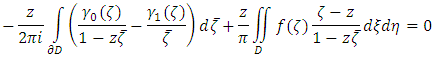and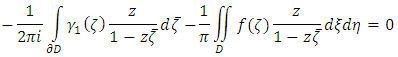the solution then is uniquely given by the formula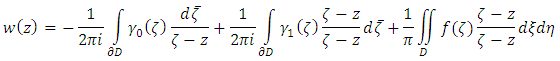As an application of Theorem 5.2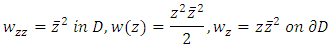The first solvability condition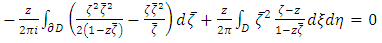(5)
and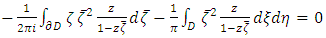(6)
then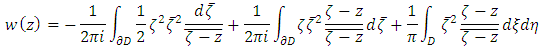By using the Gauss Theorem, the equation 5 i.e. first solvability condition can be written as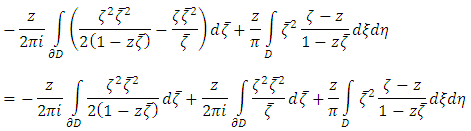And from the Gauss Theorem it follows that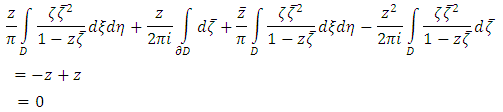Using Gauss Theorem the second solvability condition can be written as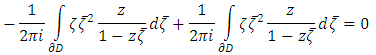So the solution is uniquely given by the following formula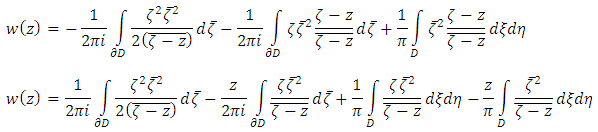Using Cauchy-Pompeiu Representation formula it follows that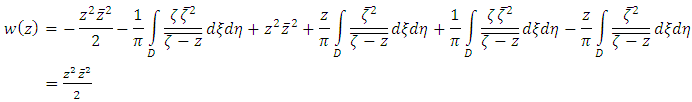Complex conjugate location of firm b is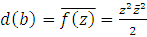, under the Dirichlet boundary condition in unit disk; we find both firms optimal location to generate equilibrium.

### 5. Conclusions

The spatial economics covers location theory, spatial competition and regional and urban economics. The fundamental problem of the spatial economics is to determine allocation of resources over space and the location of economic activity. The choice of location of economic activity directly impacts the economic growth and international trade.
In this paper, we analyzed the two-dimensional unit disk market with spatial restriction. We provide location function for the competing firms based on the market range framework in generalized Hotelling model in complex plane with boundary condition. We find the firm locations in symmetric opposite points in the plane. On the contrary to the previous studies, we consider only location game in our model to satisfy spatial restriction of the two-dimensional unit disk market. The model could be extended with inclusion of price game for further research.

### Notes

1. See 
2. Economides  used to euclidian distance metrics to find existence equilibrium.
3. Salop  intoduces cicular model on a circle with location equilibra that place firms equidistantly.
4. A market place represented as a complex space
5. It can be treated as a payoff function of firms

### References

  Anderson, S.P., Goeree, J.K., Ramer, R. (1997), Location, Location, Location, Journal of Economic Theory 77, 102-77.  Ansari, A., Economides, N., Steckel, J. (1998), The Max-Min-Min Principle of Product Differentiation. Journal of Regional Science 38, 207-230.  Ashyralyev, A., Karaca, B. (2016), A Note on The Dirichlet Problem for Model Complex Partial Differential Equations. International Conference on Analysis and Applied Mathematics, AIP Conference Proceedings Almaty, Kazakhstan 1759: 020098-1-020098-5.  Bertrand, J. (1883), Theorie mathematique de la richesse sociale. Journal des Savants 499–508.  Böckem, S. (1994), A Generalized Model of Horizontal Product Differentiation. Journal of Industrial Economics 17, 287-298.  Brenner, S. (2005), Hotteling Games with with Three, Four, and More Players. Journal of Regional Science 45, 851-864.  D’Aspremont, C., Gabszewicz, J.J., Thisse, J.-F. (1979), On Hotelling’s Stability in Competition. Econometrica 47, 1145-1150.  Economides, N. (1986), Minimal and Maximal Product Differentiation Hotteling’s Duopoly. Economics Letters 21.67-71  Feldin, A. (2012), Three Firms on a Unit Disk Market: Intermediate Product Differentiation. Economic and Business Review 14, 321-345.  Hotelling, H. (1929), Stability in Competition. Economic Journal 39, 41-57.  Irmen, A., Thisse, J.-F. (1998), Competition Multi-Characteristics Spaces: Hotteling was almost Right. Journal of Economic Theory 78, 76-102.  Lambertini, L. (1994), Equilibrium Locations in the Unconstrained Hotteling Game. Economic Notes 24, 438-446.  Karaca, B. (2016), Dirichlet problem for partial differential equations with complex variables, Ph.D. Thesis, Yeditepe University Istanbul Turkey.  Meagher, K.J., Teo, E.G.S., Wang, W. (2008), A Duopoly Toolkit: Consumer Densities which Yield Unique Spatial Duopoly Equilibria. B.E Journal of Theoretical Economics 8, 1-21.  Osborn, M.J., Pitchik, C. (1987), Equilibrium in Hotteling’s Model of Spatial Competition. Econometrica 55, 911-922.  Salop, S.C. (1979), Monopolistic Competition with Outside Goods. Bell Journal of Economics 10, 141-156.  Stadler, M. (2017), Location in a Disk City with Consumer Concentration around the Center. German Business Economic Association of Business Administration Discussion Paper Series No: 17-14, May.  Tabuchi, T. (1994), Two-Stage Two-Dimensional Spatial Competition between Two firms. Regional Science and Urban Economics 24, 207-227.  Veendorp, E. C .H., Majeed, A. (1995), Differentiation in a Two-Dimensional Market. Regional Science and Urban Economics 25, 75-83.  Woeckener, B. (2002), Spatial Competition with an Outside Good and Distributed Reservation Prices. Journal of Economics 71, 31-46.